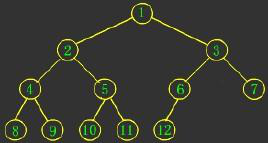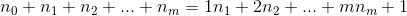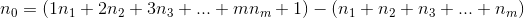# 二叉树节点数该怎么计算？有几种算法？

TheDisguiser 2020-05-28 21:51:15 java常见问答 11419n0 是叶子节点的个数

n2 是度为2的结点的个数

n0=n2+1=5+1=6

1)、程序计算法

```int leaf(bitree t)
{
if(!t)
return 0; //空树,无叶子
else if(!t->lch && !t->rch)
return 1;
else
return (leaf(t->lch) + leaf(t->rch));
}```

2)、手动计算公式3)、递归算法

```public int getTotal(Node node){
if(node == null){
return 0;
}
return getTotal(node.left) + getTotal(node.right) + 1;}```